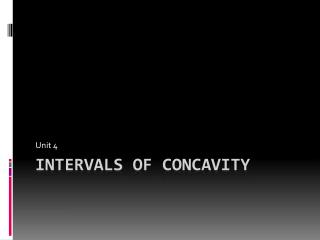DownloadDownload PresentationIntervals of Concavity

# Intervals of Concavity

Download Presentation## Intervals of Concavity

- - - - - - - - - - - - - - - - - - - - - - - - - - - E N D - - - - - - - - - - - - - - - - - - - - - - - - - - -
##### Presentation Transcript

1. Unit 4 Intervals of Concavity

2. Definition A graph is concave up if it forms a parabola that opens upward A graph is concave down if it forms a parabola that opensdownward Aninflection point is a point where a graph switches concavity

3. Example graphs Concave up Concave down

4. Example of Inflection Point Point of inflection is at (0,0). This is where the concavities change from up ward to downward. (- ) 8 8 Concave up: , 0) Concave down: ( 0, Point of Inflection: (0,0)

5. Procedure Find the second derivative Find the critical numbers Where f”(x)=0 Values of x that make f(x) or F”(x) undefined Place those values on a number line Test a value in each interval in the second derivative Concave up where f”(x) is positive Concave down where f”(x) is negative Write the intervals

6. Examples Concave up: (-1/4, Concave down: ( ,-1/4) Infection point: (-1/4, 29/8) ) 8 8 -1/4